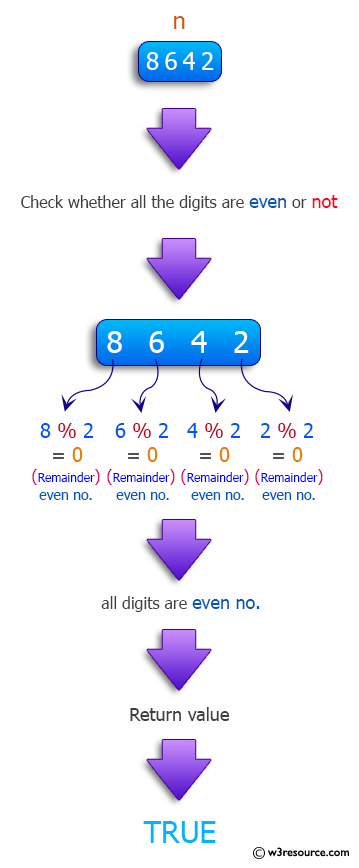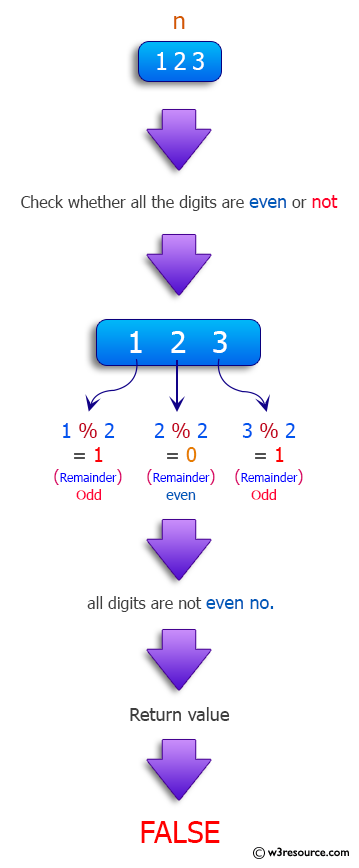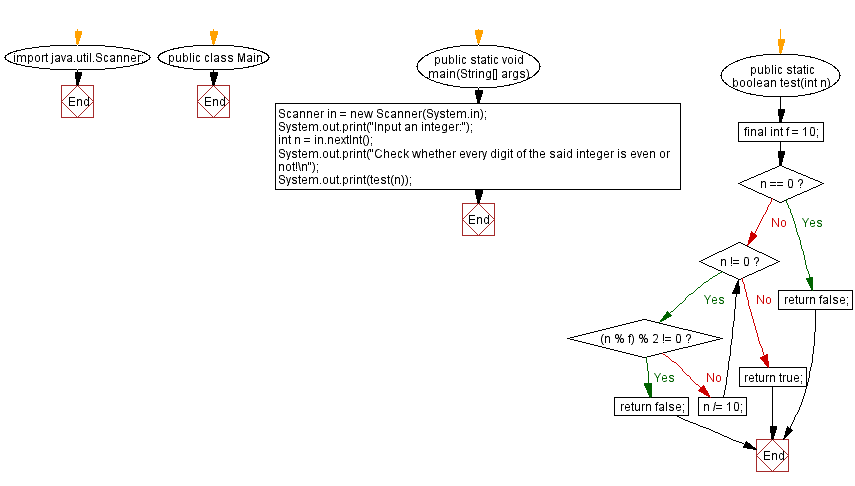﻿ Java: Check whether every digit of a given integer is even# Java Method Exercises: Check whether every digit of a given integer is even

## Java Method: Exercise-22 with Solution

Write a Java method to check whether every digit of a given integer is even. Return true if every digit is odd otherwise false.

Note: 1, 3, 5, 7, 9 are odd digits and 0, 2, 4, 6, and 8 are even digits

Sample data:
(8642)->true
(123)->false
(200)->true

Pictorial Presentation:Sample Solution:

Java Code:

``````import java.util.Scanner;
public class Main {
public static void main(String[] args)
{
Scanner in = new Scanner(System.in);
System.out.print("Input an integer:");
int n = in.nextInt();
System.out.print("Check whether every digit of the said integer is even or not!\n");
System.out.print(test(n));
}

public static boolean test(int n){
final int f = 10;
if (n == 0){
return false;
}
while(n != 0){
if((n % f) % 2 != 0){
return false;
}
n /= 10;
}
return true;
}
}
```
```

Sample Output:

```Input an integer: 8642
Check whether every digit of the said integer is even or not!
true
```

Flowchart :Java Code Editor:

Contribute your code and comments through Disqus.

Previous Java Exercise: Display the factors of 3 in a given integer.
Next Java Exercise: Check all the characters in a string are vowels or not.

What is the difficulty level of this exercise?

Test your Programming skills with w3resource's quiz.

﻿

## Java: Tips of the Day

filterNonUnique:

Filters out the non-unique values in an array.

Use Arrays.stream().filter() for an array containing only the unique values.

```public static int[] filterNonUnique(int[] elements) {
return Arrays.stream(elements)
.filter(el -> indexOf(elements, el) == lastIndexOf(elements, el))
.toArray();
}```

Ref: https://bit.ly/3CvEJ7Y